Count the number of duplicates in R

• Last Updated : 07 Apr, 2021

In this article, we will see how to find out the number of duplicates in R Programming language.

It can be done with two methods:

• Using duplicated() function.
• Using algorithm.

Method 1: Using duplicated()

Here we will use duplicated() function of R and dplyr functions.

Approach:

• Insert the “library(tidyverse)” package to the program.
• Create a data frame or a vector.
• Use the duplicated() function and check for the duplicate data.

Syntax: duplicated(x)

Parameters: x: Data frame or a vector

Example 1: Finding duplicate in vector.

Let’s first create a vector and find the position of the duplicate elements in x.

R

 x <- c(1, 1, 4, 5, 4, 6)  duplicated(x)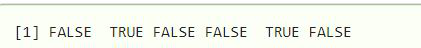Extract the duplicate elements in x.

R

 x <- c(1, 1, 4, 5, 4, 6)  duplicated(x)x[duplicated(x)]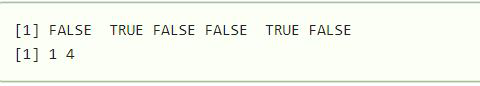Here we can see all the elements which are duplicated.

Example 2: Finding duplicate in Dataframe.

Let’s now create a data frame.

R

 data <- data.frame(  emp_id = c (1,1,2,4,5,6,6),    emp_name = c("Rick","Dan","Michelle",               "Ryan","Gary","x" , "y"))  display(data)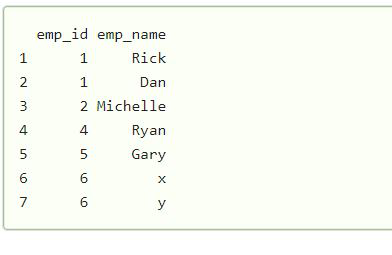Here we have a data frame and some items are duplicated, so we have to find the duplicated elements in this data frame.

We will check which column has the duplicated data.

R

 duplicated(data\$emp_id)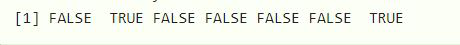So now find out in emp_id column how many duplicated elements are there.

R

 data <- data.frame(  emp_id = c (1, 1, 2, 4, 5, 6, 6),    emp_name = c("Rick", "Dan", "Michelle",               "Ryan", "Gary", "x" , "y"))  duplicated[(data\$emp_id), ]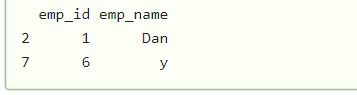We can see all the duplicated elements in column emp_id.

Method 2: Using algorithm.

Lets us assume we have a data frame with duplicate data, and we have to find out the number of duplicates in that data frame.

R

 data <- data.frame(  emp_id = c (1,1,2,4,5,6,6),    emp_name = c("Rick","Dan","Michelle","Ryan","Gary","x" , "y"))  sum(table(data\$emp_id)-1)

Output: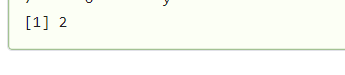We can see clearly we have calculated the number of duplicates in the data frame.

My Personal Notes arrow_drop_up